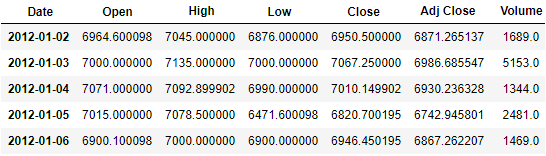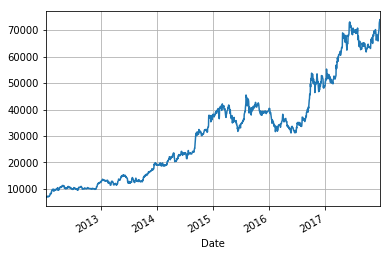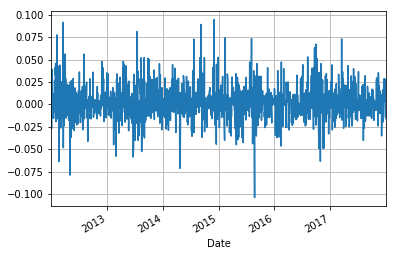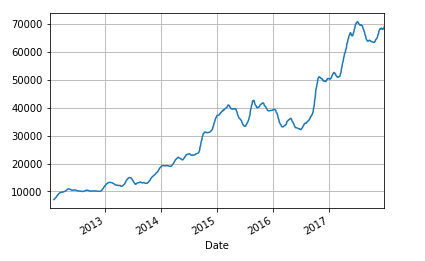# Time Series Analysis: An Introduction In Python

In this blog, we will see basic time series operations on historical stock data. Our main focus will be on generating a static forecasting model. We will also check the validity of the forecasting model by computing the mean error. However, before moving on to building the model, we will briefly touch upon some other basic parameters of time series like moving average, trends, seasonality, etc.

### Fetching Data

For the purpose of this blog, we will take past five years of ‘adjusted price’ of MRF. We will fetch this data from yahoo finance using pandas_datareader. Let us start by importing relevant libraries.

```import pandas as pd
import matplotlib.pyplot as plt
import numpy as np```

Now, let us fetch the data using DataReader. We will fetch historical data of the stock starting 1st Jan 2012 till 31st Dec 2017. You can also fetch only the adjusted closing price as this is the most relevant price, adjusted for all corporate actions and used in all financial analysis.

```stock = web.DataReader('MRF.BO','yahoo', start = "01-01-2012", end="31-12-2017")
stock = stock.dropna(how=’any’)```

We can check the data using the head() function.

`stock.head()`We can plot the adjusted price against time using the matplotlib library which we have already imported.

`stock[‘Adj Close’].plot(grid = True)`### Calculating and plotting daily returns

Using time series, we can compute daily returns and plot returns against time. We will compute the daily returns from the adjusted closing price of the stock and store in the same dataframe ‘stock’ under the column name ‘ret’. We will also plot the daily returns against time.

```stock['ret'] = stock['Adj Close'].pct_change()
stock['ret'].plot(grid=True)```#### Moving Average

Similar to returns, we can calculate and plot the moving average of the adjusted close price. Moving average is a very important metric used widely in technical analysis. For illustration purpose, we will compute 20 days moving average.

```stock['20d'] = stock['Adj Close'].rolling(window=20, center=False).mean()
stock['20d'].plot(grid=True)```

Before moving on to forecasting, let us quickly have a look on trend and seasonality in time series.#### Trend and Seasonality

In simple words, trend signifies the general direction in which the time series is developing. Trends and trend analysis are extensively used in technical analysis. If some patterns are visible in the time series at regular intervals, the data is said to have seasonality. Seasonality in a time series can impact results of the forecasting model and hence should be dealt with care.

You can refer to our blog “Starting Out with Time Series” for more details on trend and seasonality and how a time series can be decomposed into its components.

#### Forecasting

We will discuss simple linear forecasting model assuming the time series is stationary and doesn’t have seasonality. The basic assumption here is that the time series follows a linear trend. The model can be represented as:

`Forecast (t) = a + b X t`

Here 'a' is the intercept that time series makes on Y-axis and 'b' is the slope. Let us now look at the computations of a and b. Consider a time series with values D(t) for time period 't'.

In this equation, 'n' is the sample size. We can validate our model by calculating the forecasted values of D(t) using the above model and comparing the values against actual observed values. We can compute mean error which is the mean value of the difference between the forecasted D(t) and the actual D(t).

In our stock data, D(t) is the adjusted closing price of MRF. We will now compute the values of a, b, forecasted values and their errors using python.

```#Populates the time period number in stock under head t
stock['t'] = range (1,len(stock)+1)
#Computes t squared, tXD(t) and n
stock['sqr t']=stock['t']**2
n=len(stock)
#Computes slope and intercept
slope = (n*stock['tXD'].sum() - stock['t'].sum()*stock['Adj Close'].sum())/(n*stock['sqr t'].sum() - (stock['t'].sum())**2)
intercept = (stock['Adj Close'].sum()*stock['sqr t'].sum() - stock['t'].sum()*stock['tXD'].sum())/(n*stock['sqr t'].sum() - (stock['t'].sum())**2)
print ('The slope of the linear trend (b) is: ', slope)
print ('The intercept (a) is: ', intercept)```

The above code gives the output as shown.

```The slope of the linear trend (b) is: 41.2816591061

The intercept (a) is: 1272.6557803```

We can now check the validity of our model by computing the forecasted values and computing the mean error.

```#Computes the forecasted values
stock['forecast'] = intercept + slope*stock['t']
#Computes the error
stock['error'] = stock['Adj Close'] - stock['forecast']
mean_error=stock['error'].mean()
print ('The mean error is: ', mean_error)```

The mean error is given by the output as:

`The mean error is: 1.0813935108094419e-10`

As can be seen from the above value of the mean error, our model gave results very close to the actual values. Hence, the data is free from any seasonality.

### Summary

In this blog, we have seen various properties of time series and how to compute these properties in python. We have also seen a simple model to forecast our time series based on trend assuming that the time series is free from seasonality.

### Next Step

Read our post on 'Time Series Analysis: Working With Date-Time Data In Python' that focuses on dealing with dates and frequency of the time series and performing Time Series Analysis in python by extensively using the date time library.

Disclaimer: All investments and trading in the stock market involve risk. Any decisions to place trades in the financial markets, including trading in stock or options or other financial instruments is a personal decision that should only be made after thorough research, including a personal risk and financial assessment and the engagement of professional assistance to the extent you believe necessary. The trading strategies or related information mentioned in this article is for informational purposes only.

Update

We have noticed that some users are facing challenges while downloading the market data from Yahoo and Google Finance platforms. In case you are looking for an alternative source for market data, you can use Quandl for the same.

``````
``````